# CalcKit: All-In-One Calculator

CalcKit is a simple but powerful collection of calculators and converters for math, electronics, finance, and more.

Designed with simplicity in mind, it helps you solve all kinds of everyday problems.

From simple calculations to unit and currency conversions, percentages, proportions, areas, volumes, etc… it does it all.

Featuring over 150 FREE Calculators and Unit Converters, packed with a highly customizable Scientific Calculator, it's the only calculator you'll need on your device from now on.

Scientific Calculator
★ Instant results
★ Copy and paste support
★ Editable input & cursor
★ Memory buttons
★ Floating calculator widget

150 Calculators & Converters
★ Algebra, Geometry, Electronics, Finance, Unit Converters & Misc
★ Currency converter with 180 currencies and crypto, available offline
★ Instant results as you type
★ Smart search for faster navigation
★ Create shortcuts on home screen

Custom Calculators
★ Custom tools builder for creating your own calculators
★ Up to 25 variables per calculator
★ Detailed tutorial with examples

Algebra
• Percentage calculator
• Proportion calculator
• Average calculator - arithmetic, geometric and harmonic means
• Equation solver - linear, quadratic, cubic and equation systems
• Combinations and permutations
• Decimal to fraction
• Fraction simplifier
• Prime number checker
• Greatest common factor & Least common multiple
• Matrix calculator

Geometry
• Triangle, right triangle, square, rectangle, parallelogram, rhombus, trapezoid, hexagon, polygon, circle, circular segment, circular sector, ellipse
• Cube, cuboid, prism, pyramid, pyramidal frustum, tetrahedron, octahedron, cylinder, truncated cylinder, cone, conical frustum, sphere, spherical cap, spherical sector, spherical segment, spherical wedge, torus

Electronics
• Resistor color code - up to 6 bands
• Inductor color code
• LED resistor calculator
• Series and parallel components - resistors, capacitors, inductors
• Ohm's law
• Power triangle
• Y-Δ Transformation
• Voltage divider
• Voltage regulator - LM317
• Operational amplifier
• NE555 timer calculator
• Filters calculator
• Reactance and resonance calculator
• Wire resistance and conductance
• Transformer ratio
• Battery life calculator
• Analog-Digital converter
• Frequency calculator

Finance
• Currency converter with 180 currencies and crypto, available offline
• Sales tax calculator
• Tip calculator
• Simple and compound interest calculator

Unit Converters
• Angle converter
• Area converter
• Cooking converter
• Data storage converter
• Energy converter
• Force converter
• Fuel converter
• Length converter
• Weight converter
• Numeric base converter
• Power converter
• Prefixes converter
• Pressure converter
• Roman numerals converter
• Shoe size converter
• Speed converter
• Temperature converter
• Time converter
• Volume converter
• Acceleration converter
• Airflow converter
• Density converter
• Inertia converter
• Torque converter
• Voltage converter
• Current converter
• Charge converter
• Conductance converter
• Field strength converter
• Resistance converter
• Resistivity converter
• Capacitance converter
• Inductance converter
• Flow converter
• Flux converter
• Permeability converter
• Viscosity converter
• Heat converter
• Light converter
• Magnetism converter
• Frequency converter
• and more…

Miscellaneous
• Date calculator
• Time calculator
• Analytical geometry - distance between 2 points, area of triangle, equation of circle and equation of sphere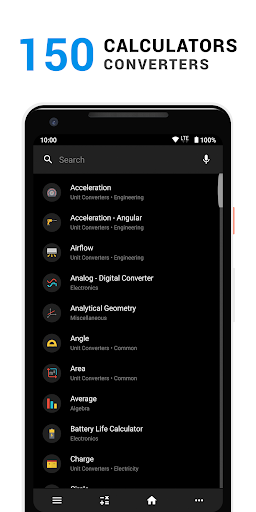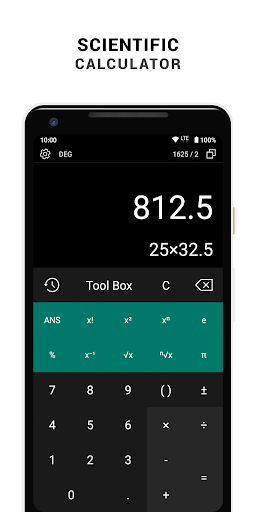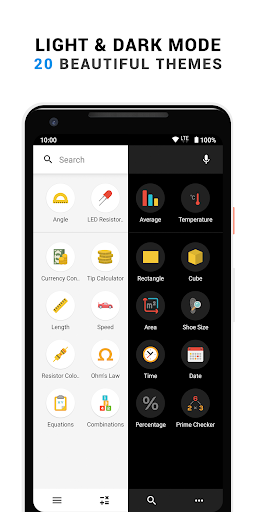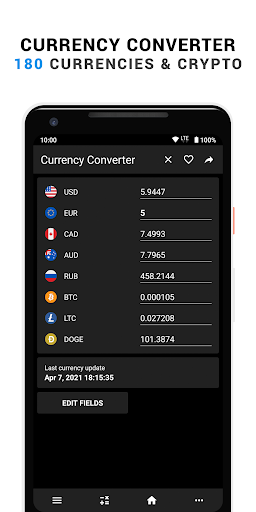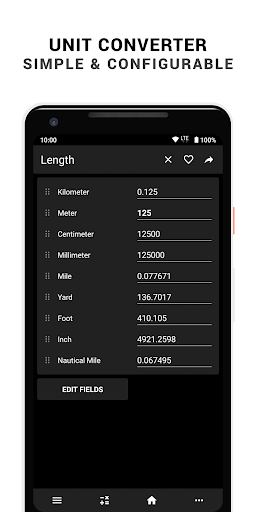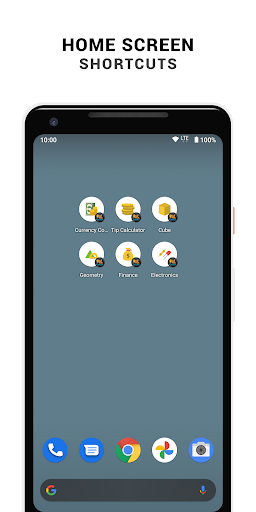##### Recent Updates - Version 5.0.0
• CalcKit v5.0.0

• Released the first official stable version of the CalcKit Web App.
• Added 4 new Finance calculators
• Improved Interest calculator
• Added the option to create and restore full backups of CalcKit
• Added 6 new tools to the Community Library